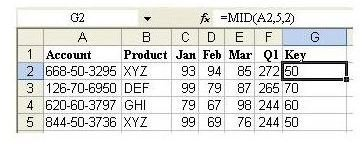# Microsoft Excel: How to Isolate the Middle Portion of a Cell with MID Function

Page content

## Using the MID Function to Extract Middle Values

Excel’s MID function is a powerful tool that lets you isolate and extract the contents in the middle of a cell. The following example illustrates how to use this tool by using the MID function to extract the values from the middle of an account number and displaying them in a new column.

Strategy: Insert a new column and use the MID function to isolate the necessary digits from the account field.

The MID function takes three arguments. The first argument is a cell containing a text value. The second argument is the character number where you want the result to start. The final argument is the length of the result.

In a well-formed account number, like 123-45-6789, you can predict that the start of the second segment will always be in the fifth character position. (The “-” counts as a character here.) The length of the second segment is always two characters.

Click any image for a larger view.

In a blank column, enter a heading, such as the word “Key” – or anything else that makes sense for your particular table. In cell G2, enter the formula =MID(A2,5,2). Copy the formula down to all rows, as shown in Fig. 267.Additional Details: In order to capture the final four digits of the account number, you could either use the =MID(A2,8,4) or the =RIGHT(A2,4) function to isolate the final four digits of the account number.

Result: You can now sort by the new column and add subtotals by this field.

Summary: When you need to isolate a portion of the characters in another column, creating a temporary column and using the MID function is the solution.

Functions Discussed: =MID(); =RIGHT()

Cross Reference: How to Sort on One Segment of an Account ID